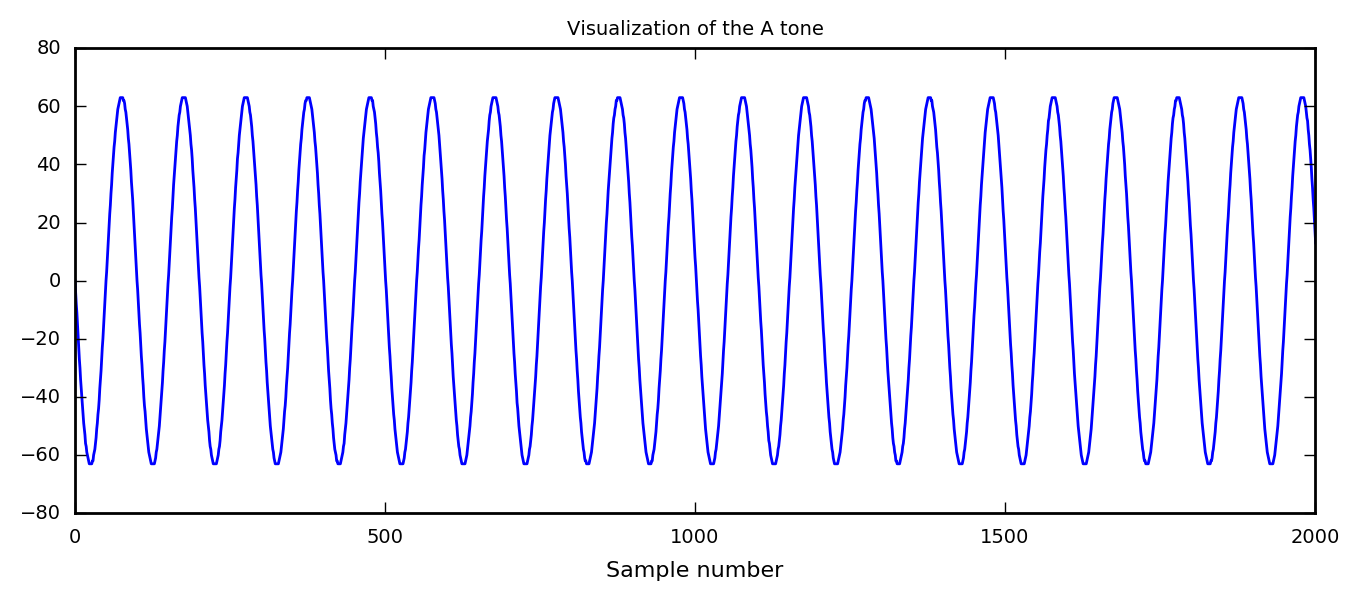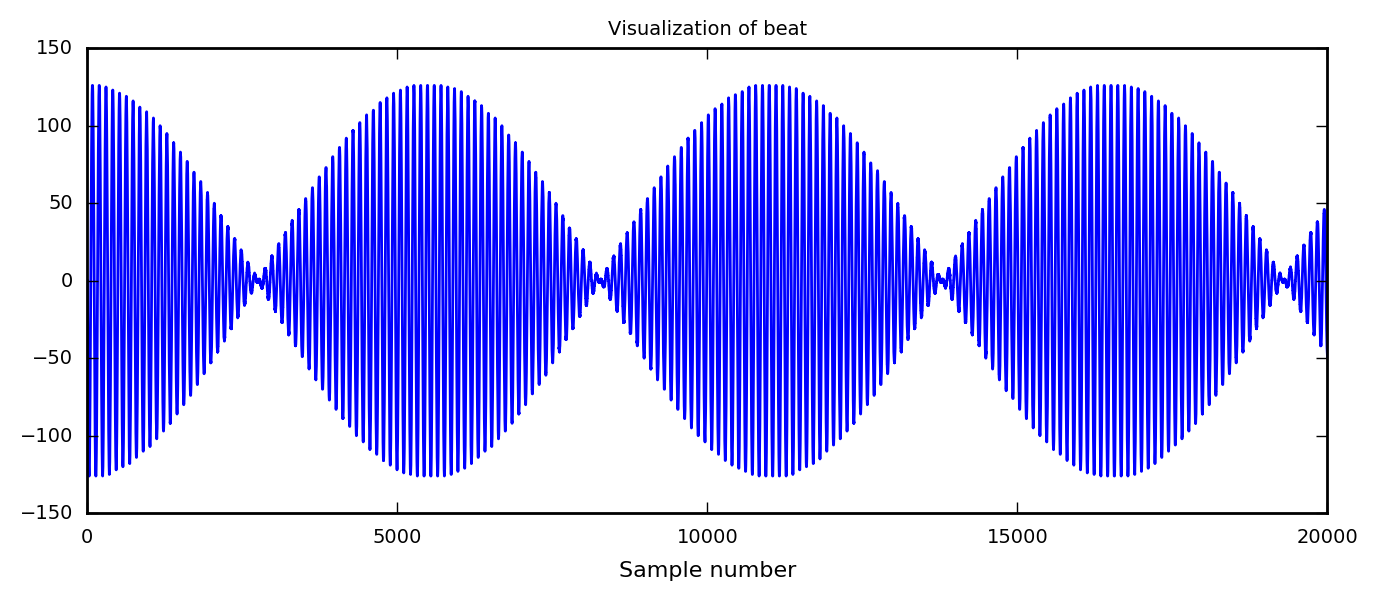# Simple Sound Filtering using Discrete Fourier Transform¶

### Example – Waves and Acoustics¶

By Jonas Tjemsland, Andreas Krogen, Håkon Ånes og Jon Andreas Støvneng.

Last edited: May 20th 2016

This notebook gives an introduction to programming with sounds in Python. We are using Python's wave class. Check it out to see how the different functions are operated! Towards the end, a couple of sound tracks are filtered using discrete Fourier transforms (DFTs) and the trigonometric least squares approximation.

NOTE: Some of the sound files might be a bit loud, and you are adviced to turn down the volume before you play them.

As always, we start by importing necessary libraries, and set common figure parameters.

In :
import numpy as np
from matplotlib import pylab as plt
import wave
import IPython
from scipy import fftpack as fft
%matplotlib inline

# Casting unitary numbers to real numbers will give errors
# because of numerical rounding errors. We therefore disable
# warning messages.
import warnings
warnings.filterwarnings('ignore')

# Set common figure parameters
newparams = {'axes.labelsize': 8, 'axes.linewidth': 1, 'savefig.dpi': 200,
'lines.linewidth': 1, 'figure.figsize': (8, 3),
'ytick.labelsize': 7, 'xtick.labelsize': 7,
'legend.fontsize': 7, 'legend.frameon': True,
'legend.handlelength': 1.5, 'axes.titlesize': 7,}
plt.rcParams.update(newparams)


### Programming with sound¶

A sound is a longitudinal wave in a medium, such as air or water. It is a vibration that propagates through the medium as fluctuations of pressure and displacements of particles. For example, the A tone is a sound wave of frequency 440 Hz, created by a tuning fork, speaker, string or another device which makes the air oscillate with the given frequency. On a computer, the sounds are nothing but a sequence of numbers. A given tone can mathematically be described by

$$s(t)=A\sin(2\pi f t),$$

where $A$ is the amplitude, $f$ is the frequency and $t$ is time. In a computer, $s(t)$ is evaluated at discrete points in time, determined by the sampling rate. An ordninary audio CD has a sampling frequency of 44100 Hz. Sampling will be discussed below.

The following function creates a tune with specific frequency, length, amplitude and sampling rate.

In :
def tone(frequency=440., length=1., amplitude=1., sampleRate=44100., soundType='int8'):
""" Returns a sine function representing a tune with a given frequency.

:frequency: float/int. Frequency of the tone.
:length: float/int. Length of the tone in seconds.
:amplitude: float/int. Amplitude of the tone.
:sampleRate: float/int. Sampling frequency.
:soundType: string. Type of the elements in the returned array.
:returns: float numpy array. Sine function representing the tone.
"""
t = np.linspace(0,length,length*sampleRate)
data = amplitude*np.sin(2*np.pi*frequency*t)
return data.astype(soundType)


For simplicity, let us create a nostalgic 8 bit mono wav sound file. Note that 8 bit samples are stored as uint8, ranging from 0 to 255, while 16-bit samples are stored as int16, ranging from -32768 to 32767. In other words, since we are creating an audiofile of samplewidth 8 bits, we need to add 128 to the samples before we write to file. The wave module will automatically change to uint8 if this is not done, such that -128 becomes 128, -127 becomes 129, and so on, and the sound file may become somewhat distored. Thanks to Øystein Hiåsen for pointing this out! If we where to use 16-bit samples we don't have to worry about this. This notebook supports 8, 16 and 32 bit samples.

In :
# Parameters that are being used in the start of this notebook
sampleRate = 44100
sampwidth = 1          # In bytes. 1 for 8 bit, 2 for 16 bit and 4 for 32 bit
volumePercent = 50     # Volume percentage
nchannels = 1          # Mono. Only mono works for this notebook

# Some dependent variables
shift = 128 if sampwidth == 1 else 0 # The shift of the 8 bit samples, as explained in the section above.
soundType = 'i' + str(sampwidth)
amplitude = np.iinfo(soundType).min*volumePercent/100.

In :
# Parameters for a A tone lasting 1 second at a sample
frequency = 440
length = 1

# Calculate the sine function for the given parameters
data = tone(frequency, length, amplitude, sampleRate, soundType)

# Plot the function
plt.plot(data)
plt.xlim([0,2000])
plt.title('Visualization of the A tone')
plt.xlabel('Sample number')

# Open a new .wav-file in "write" mode, set file parameters
# and write to file
data += shift # Shift the samplings if we use 8 bit
with wave.open('Atone.wav', 'w') as file:
file.setparams((nchannels, sampwidth, sampleRate, 0, 'NONE', ''))
file.writeframes(data)

# Display the sound file
IPython.display.Audio('Atone.wav')

Out:Let us try to create a beat by letting two waves of different frequency interfere. The beat will then have a frequency equal to the absolute value of the difference in frequency of the two waves, $f = \left|f_2 - f_1\right|$. You can read more about beat frequencies on the great Hyperphysics website.

In :
frequency = 400
frequency2 = 408
length = 5

# Calculate the sine function for the given parameters
data = ( tone(frequency, length, amplitude, sampleRate,soundType) +
tone(frequency2, length, amplitude, sampleRate,soundType) )

# Plot the function
plt.plot(data)
plt.xlim([0,20000])
plt.title('Visualization of beat')
plt.xlabel('Sample number')

# Create sound file
data += shift
with wave.open('beat.wav','w') as file:
file.setparams((nchannels, sampwidth, sampleRate, 0, 'NONE', ''))
file.writeframes(data)

IPython.display.Audio('beat.wav')

Out:Using these concepts, we can actually create a simple melody.

In :
# Create a "function" to translate from a given tone to its frequency
baseTone = 130.813  # The frequecy of the tone C3, bass C
tones = ['C', 'C#', 'D', 'D#', 'E', 'F', 'F#', 'G', 'G#', 'A', 'Bb', 'B',
'Ch','C#h','Dh','D#h','Eh','Fh','F#h','Gh','G#h','Ah','Bbh','Bh']
# And use dictionary comprehension to fill in the frequencies
notes2freq = {tones[i]: baseTone*2**(i/12) for i in range(0,len(tones))}

# The meldody data saved as a list of tuples (note, duration)
l = 2.
notes = [('D',0.083*l),('D',0.083*l),('D',0.083*l),('G',0.5*l),('Dh',0.5*l),('Ch',0.083*l),
('B',0.083*l),('A',0.083*l),('Gh',0.5*l),('Dh',0.25*l),('Ch',0.083*l),('B',0.083*l),
('A',0.083*l),('Gh',0.5*l),('Dh',0.25*l),('Ch',0.083*l),('B',0.083*l),('Ch',0.083*l),
('A',0.5*l),('D',0.167*l),('D',0.083*l),('G',0.5*l),('Dh',0.5*l),('Ch',0.083*l),
('B',0.083*l),('A',0.083*l),('Gh',0.5*l),('Dh',0.25*l),('Ch',0.083*l),('B',0.083*l),
('A',0.083*l),('Gh',0.5*l),('Dh',0.25*l),('Ch',0.083*l),('B',0.083*l),('Ch',0.083*l),
('A',0.5*l),('D',0.167*l),('D',0.083*l),('E',0.375*l),('E',0.125*l),('Ch',0.125*l),
('B',0.125*l),('A',0.125*l),('G',0.125*l),('G',0.083*l),('A',0.083*l),('B',0.083*l),
('A',0.167*l),('E',0.083*l),('F#',0.25*l),('D',0.167*l),('D',0.083*l),('E',0.375*l),
('E',0.125*l),('Ch',0.125*l),('B',0.125*l),('A',0.125*l),('G',0.125*l),
('Dh',0.1875*l),('A',0.0625*l),('A',0.5*l),('D',0.167*l),('D',0.083*l),('E',0.375*l),
('E',0.125*l),('Ch',0.125*l),('B',0.125*l),('A',0.125*l),('G',0.125*l),('G',0.083*l),
('A',0.083*l),('B',0.083*l),('A',0.167*l),('E',0.083*l),('F#',0.25*l),('Dh',0.167*l),
('Dh',0.083*l),('Gh',0.125*l),('Fh',0.125*l),('D#h',0.125*l),('Ch',0.125*l),
('Bb',0.125*l),('A',0.125*l),('G',0.125*l),('Dh',0.75*l)]

# Create the file data
data = np.array([],dtype=soundType)
for note, duration in notes:
currentFrequency = notes2freq[note]
currentTone = tone(currentFrequency, duration, amplitude, sampleRate, soundType)
data = np.append(data, currentTone)
data += shift
with wave.open('melody.wav','w') as file:
file.setparams((nchannels, sampwidth, sampleRate, 0, 'NONE', ''))
file.writeframes(data)

IPython.display.Audio('melody.wav')

Out: